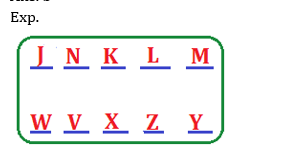# RRB PO Prelims Reasoning Ability Quiz – 6

## RRB PO Prelims Reasoning Ability Quiz

Reasoning Ability plays a very crucial role in every competitive examination. With consistent practice, candidates can ace this section in examination. In this article, we bring to you RRB PO Reasoning Ability Quiz to boost your preparation. This RRB PO Reasoning Ability Quiz contains various types of questions ranging from easy to difficult level. This RRB PO Reasoning Ability Quiz is absolutely FREE. Candidates will be provided with a detailed explanation of each question in this RRB PO Reasoning Ability Quiz. Question given in this RRB PO Reasoning Ability Quiz are based on the latest or updated exam pattern. In order to be able to answer questions quickly and efficiently in upcoming exams, aspirants must practice this RRB PO Reasoning Ability Quiz.

Directions (1-5): Study the given information carefully to answer the given questions:
Ten persons are sitting in two parallel rows containing five persons each, in such a way that there is an equal distance between adjacent persons. In row —1, J, K, L, M, and N are seated (not necessarily in the same order) and all of them are facing south. In row-2, V, W, X, Y and Z are seated (not necessarily in the same order) and all of them are facing north. Therefore in the given seating arrangement each member seated in row faces another member of the other row.
Z sits third to the right of W. V sits second to the left of Z. The person facing V sits to the immediate right of K. Only one person sits between K and M. J is not an immediate neighbour of K. Only two persons sit between J and L. Neither K nor J faces Y.

1. Who amongst the following is facing N?
(a) Y
(b) V
(c) X
(d) W
(e) Z

Ans. b2. Which of the following statements is true regarding M?
(a) M faces one of the immediate neighbours of X.
(b) K is one of the immediate neighbours of M.
(c) None of the given statements is true.
(d) L sits to the immediate right of M.
(e) Only one person sits between M and N.

Ans. d3. Who amongst the following is facing X?
(a) K
(b) L
(c) M
(d) J
(e) N

Ans. a4. What is the position of Z with respect of Y?
(a) Third to the right
(b) second to the right
(c) Immediate left
(d) Immediate right
(e) Second to the left

Ans. c5. Four of the following five are alike in a certain way based on the given arrangement and hence form a group. Which of them does not belong to that group?
(a) M
(b) J
(c) Y
(d) W
(e) N

Ans. eDirections (6-10): In these questions, relationship between different elements is shown in the statements. The statements are followed by conclusions. Study the conclusions based on the given statements and select the appropriate answer. Give answer-
6. Statements: S ≤ L ≤ I = P ≥ E > R; L > Q
Conclusions: I. P ≥ S II. I > R
(a) If only conclusion II is true
(b) If only conclusion I is true
(c) If both conclusions I and II are true.
(d) If either conclusion I or II is true
(e) If neither conclusion I nor II is true.

Ans. c
Exp. I. P ≥ S (True) II. I > R (True)

7. Statements: G > R ≥ E = A ≤ T ≤ S; D ≤ A ≤ J
Conclusions: I. T ≥ D II. R > S
(a) If only conclusion II is true
(b) If only conclusion I is true
(c) If both conclusions I and II are true.
(d) If either conclusion I or II is true
(e) If neither conclusion I nor II is true.

Ans. b
Exp. I. T ≥ D (True) II. R > S (False)

8. Statements: A ≥ B > C ≤ D ≤ E < F
Conclusions: I. A ≥ E II. C < F
(a) If only conclusion II is true
(b) If only conclusion I is true
(c) If both conclusions I and II are true.
(d) If either conclusion I or II is true
(e) If neither conclusion I nor II is true.

Ans. a
Exp. I. A ≥ E (False) II. C < F (True)

9. Statements: G > R ≥ E = A ≤ T ≤ S; D ≤ A ≤ J
Conclusions: I. J > G II. J = G
(a) If only conclusion II is true
(b) If only conclusion I is true
(c) If both conclusions I and II are true.
(d) If either conclusion I or II is true
(e) If neither conclusion I nor II is true.

Ans. e
Exp. I. J > G (False) II. J = G (False)

10. Statements: S ≤ L ≤ I = P ≥ E > R; L > Q
Conclusions: I. L < R II. E ≥ Q
(a) If only conclusion II is true
(b) If only conclusion I is true
(c) If both conclusions I and II are true.
(d) If either conclusion I or II is true
(e) If neither conclusion I nor II is true.

Ans. e
Exp. I. L < R (False) II. E ≥ Q (False)### Preparation Kit PDF

#### Most important PDF’s for Bank, SSC, Railway and Other Government Exam : Download PDF Now

AATMA-NIRBHAR Series- Static GK/Awareness Practice Ebook PDF Get PDF here
The Banking Awareness 500 MCQs E-book| Bilingual (Hindi + English) Get PDF here
AATMA-NIRBHAR Series- Banking Awareness Practice Ebook PDF Get PDF here
Computer Awareness Capsule 2.O Get PDF here
AATMA-NIRBHAR Series Quantitative Aptitude Topic-Wise PDF 2020 Get PDF here
Memory Based Puzzle E-book | 2016-19 Exams Covered Get PDF here
Caselet Data Interpretation 200 Questions Get PDF here
Puzzle & Seating Arrangement E-Book for BANK PO MAINS (Vol-1) Get PDF here

3